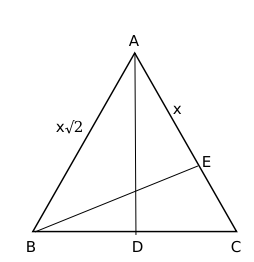Question 49

# In triangle ABC, altitudes AD and BE are drawn to the corresponding bases. If $$\angle BAC = 45^{\circ}$$ and $$\angle ABC=\theta\$$, then $$\frac{AD}{BE}$$ equals

SolutionIt is given, Angle BAE = 45 degrees

This implies AE = BE

Let AE = BE = x

In right-angled triangle ABD, it is given $$\angle ABC=\theta\$$

$$\sin\theta=\frac{AD}{AB}\$$

$$\sin\theta=\frac{AD}{x\sqrt{\ 2}}\$$

$$\sqrt{\ 2}\sin\theta=\frac{AD}{BE}\$$